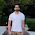## Anomaly Detection -

Anomalies are present in many industrial and non-industrials application. Intrusion detection, fraud prevention, identifying issue in any running industrial device and some illness identification, all these require some kind of anomaly detection. From machine learning perspective there are 3 types of anomaly detection techniques-

### Types of Anomaly Detection-

1. Unsupervised Anomaly detection – Some clustering algorithms like K-means are used to do unsupervised anomaly detection. Here all the features are passed to clustering algorithm and outliers are treated as abnormal data points.

2. Semi Supervised Anomaly detection technique - In this approach, a normal model aka relation among the features is prepared and treated as ideal model. An electric motor might have thermal abnormalities. So a regression or complex relationship is established between current and  temperature. Let’s say a neural network is used to fit this relation and temperature is forecasted using current. At run time actual values of temperature should match with forecasting value of temperature. The relationship that we got from NN should remain the same. Higher the error, higher chances of having abnormalities in the system.

3. Supervised Anomaly detection techniques- These are used when abnormalities are known in training period. These can be solved using classification techniques like decision tree. For example, a water pump is bent, this is known abnormality so characteristics of system (water pressure, temp, electricity used etc) at bent pipe is different from normal running pipe. If one classifies this data, he can get rules and these rules can be used to identify abnormal condition in future.

Unsupervised techniques require to build algorithm every time we want to identify abnormalities while other two requires building model just once. A combination of supervised and unsupervised is also used some times when output from the unsupervised detection is converted into classification data and then get the rules by running classification on same data. This avoids problem of building model every time.

### Anomaly Detection by Distance and Density Based Algorithm

Anomaly Detection using K-Means Clustering – This is a type of distance based unsupervised anomaly detection technique-
## subsetting IRIS data-
iris2 <- iris[,1:4]

# running K means clustering
kmeans.result <- kmeans(iris2, centers=3)
plot(iris2[,c("Sepal.Length", "Sepal.Width")], pch=19, col=kmeans.result\$cluster, cex=1)
centers <- kmeans.result\$centers[kmeans.result\$cluster, ] # "centers" is a data frame of 3 centers but the length of iris

# distance from the respective center
distances <- sqrt(rowSums((iris2 - centers)^2))
outliers <- order(distances, decreasing=T)[1:5]

## plotting outliers+ centers and all data points
print(outliers) # these rows are 5 top outliers
points(kmeans.result\$centers[,c("Sepal.Length", "Sepal.Width")], col=1:3, pch=15, cex=2)
points(iris2[outliers, c("Sepal.Length", "Sepal.Width")], pch="+", col=4, cex=3)

Anomaly Detection Using Local Outlier Factor (LOF)- Local outlier factor is more useful when there are multiple operating conditions for the system. Due to the local approach, LOF is able to identify outliers in a data set that would not be outliers in another area of the data set. For example, a point at a "small" distance to a very dense cluster is an outlier, while a point within a sparse cluster might exhibit similar distances to its neighbors. It is nicely explained here- LOF

#Sub-setting the data
iris2 <- iris[,1:4]

k <- 5 # number of neighbours
library(DMwR)

# running LOF Code
outlier.scores <- lofactor(iris2, k)

# taking data points with high LOF score only
iris2\$LOF_Score <- outlier.scores
iris3 <- iris2[order(iris2\$LOF_Score, decreasing = T),]

# subsetting and plotting the data
iris4 <- iris3[, c("Sepal.Length", "Sepal.Width")]
plot(iris4)
lof_outlier <- iris4[c(1:5),]
points(lof_outlier,pch="+", col=4, cex=3)

Here we can see that LOF has identified local outliers while K-means collects global outlier. K-means might collect outliers from less dense cluster while LOF try to give equal weight by diving the readability distance of a points by it's neighbor's readability.

Other complex outlier detection techniques-

Angle based outlier detection method

Connectivity based outlier detection methid

read about the Hierarchical Clustering (Bottom-Up Clustering) & Performance Parameters if you are looking for some lucid explanation and difference between both the type of clustering.

basics of statistics topics - Graphical explanation of Linear regression equations.  Assumptions of LR- (Graphical Explanation)

1.1.